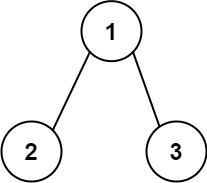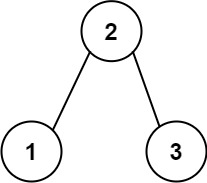### LeetCode• ㊗️
• 大家
• offer
• 多多！

## Problem

Given the root of a binary tree, return the length of the longest consecutive path in the tree.

A consecutive path is a path where the values of the consecutive nodes in the path differ by one. This path can be either increasing or decreasing.

• For example, [1,2,3,4] and [4,3,2,1] are both considered valid, but the path [1,2,4,3] is not valid.

On the other hand, the path can be in the child-Parent-child order, where not necessarily be parent-child order.

Example 1:Input: root = [1,2,3]
Output: 2
Explanation: The longest consecutive path is [1, 2] or [2, 1].


Example 2:Input: root = [2,1,3]
Output: 3
Explanation: The longest consecutive path is [1, 2, 3] or [3, 2, 1].


Constraints:

• The number of nodes in the tree is in the range [1, 3 * 10^4].
• -3 * 10^4 <= Node.val <= 3 * 10^4

## Code

298. Binary Tree Longest Consecutive Sequence

543. Diameter of Binary Tree

class Solution {
int max = 0;

public int longestConsecutive(TreeNode root) {
helper(root);
return max;
}

public int[] helper(TreeNode root){
if(root == null) return new int[]{0, 0};

int[] left = helper(root.left);
int[] right= helper(root.right);

int inc = 1;
int dec = 1;

if(root.left != null){
if(root.val - root.left.val == 1){
inc = left + 1;
}else if(root.val - root.left.val == -1){
dec = left + 1;
}
}

if(root.right != null){
if(root.val - root.right.val == 1){
inc = Math.max(inc, right + 1);
}else if(root.val - root.right.val == -1){
dec = Math.max(dec, right + 1);
}
}

max = Math.max(max, inc + dec - 1);

return new int[]{inc, dec};
}
}